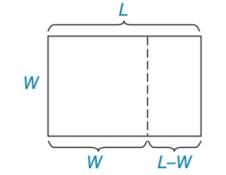Chapter 5.1, Problem 38EElementary Geometry For College St...

7th Edition
Alexander + 2 others
ISBN: 9781337614085

Solutions

Chapter
SectionElementary Geometry For College St...

7th Edition
Alexander + 2 others
ISBN: 9781337614085
Textbook Problem

To find the golden ratio (see the Discover activity on page 227), solve the equation 1 L = L − 1 L for L.(HINT: You will need the Quadratic Formula.)To determine

To find:

The solution of L.

Explanation

According to the quadratic formula, the solutions of ax2 + bx + c = 0 are given by

x=b+b24ac2a,bb24ac2a

Calculation:

Consider, 1L=L11

L(L1)=11L2L=1L2L1=0

Use the quadratic formula in L2L1=0

Still sussing out bartleby?

Check out a sample textbook solution.

See a sample solution

The Solution to Your Study Problems

Bartleby provides explanations to thousands of textbook problems written by our experts, many with advanced degrees!

Get Started

27-30 Find y and y. y=lnx1+lnx

Calculus (MindTap Course List)

Evaluate the integral. 29. 0xsinxcosxdx

Single Variable Calculus: Early Transcendentals

True or False: dmdv stands for the derivative of the function m with respect to the variable v.

Study Guide for Stewart's Single Variable Calculus: Early Transcendentals, 8th

True or False: is a convergent series.

Study Guide for Stewart's Multivariable Calculus, 8th